The Graphics class contains a set of methods that you can use to create a vector shape. Display objects that support drawing include Sprite and Shape objects. Each of these classes includes a `graphics` property that is a Graphics object. The following are among those helper functions provided for ease of use: `drawRect()`, `drawRoundRect()`, `drawCircle()`, and `drawEllipse()`.

You cannot create a Graphics object directly from ActionScript code. If you call `new Graphics()`, an exception is thrown.

The Graphics class is final; it cannot be subclassed.

### `@:value({ smooth : false, repeat : true, matrix : null })beginBitmapFill(bitmap:BitmapData, ?matrix:Matrix, repeat:Bool = true, smooth:Bool = false):Void`

Fills a drawing area with a bitmap image. The bitmap can be repeated or tiled to fill the area. The fill remains in effect until you call the `beginFill()`, `beginBitmapFill()`, `beginGradientFill()`, or `beginShaderFill()` method. Calling the `clear()` method clears the fill.

The application renders the fill whenever three or more points are drawn, or when the `endFill()` method is called.

Parameters:

`bitmap` A transparent or opaque bitmap image that contains the bits to be displayed. A matrix object(of the openfl.geom.Matrix class), which you can use to define transformations on the bitmap. For example, you can use the following matrix to rotate a bitmap by 45 degrees(pi/4 radians): ``````matrix = new openfl.geom.Matrix(); matrix.rotate(Math.PI / 4); ``` `````` If `true`, the bitmap image repeats in a tiled pattern. If `false`, the bitmap image does not repeat, and the edges of the bitmap are used for any fill area that extends beyond the bitmap. For example, consider the following bitmap(a 20 x 20-pixel checkerboard pattern):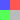When `repeat` is set to `true`(as in the following example), the bitmap fill repeats the bitmap: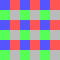When `repeat` is set to `false`, the bitmap fill uses the edge pixels for the fill area outside the bitmap: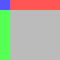If `false`, upscaled bitmap images are rendered by using a nearest-neighbor algorithm and look pixelated. If `true`, upscaled bitmap images are rendered by using a bilinear algorithm. Rendering by using the nearest neighbor algorithm is faster.

### `@:value({ alpha : 1, color : 0 })beginFill(color:Int = 0, alpha:Float = 1):Void`

Specifies a simple one-color fill that subsequent calls to other Graphics methods(such as `lineTo()` or `drawCircle()`) use when drawing. The fill remains in effect until you call the `beginFill()`, `beginBitmapFill()`, `beginGradientFill()`, or `beginShaderFill()` method. Calling the `clear()` method clears the fill.

The application renders the fill whenever three or more points are drawn, or when the `endFill()` method is called.

Parameters:

`color` The color of the fill(0xRRGGBB). The alpha value of the fill(0.0 to 1.0).

### `@:value({ focalPointRatio : 0, interpolationMethod : InterpolationMethod.RGB, spreadMethod : SpreadMethod.PAD, matrix : null })beginGradientFill(type:GradientType, colors:Array<Int>, alphas:Array<Float>, ratios:Array<Int>, ?matrix:Matrix, spreadMethod:SpreadMethod = SpreadMethod.PAD, interpolationMethod:InterpolationMethod = InterpolationMethod.RGB, focalPointRatio:Float = 0):Void`

Specifies a gradient fill used by subsequent calls to other Graphics methods(such as `lineTo()` or `drawCircle()`) for the object. The fill remains in effect until you call the `beginFill()`, `beginBitmapFill()`, `beginGradientFill()`, or `beginShaderFill()` method. Calling the `clear()` method clears the fill.

The application renders the fill whenever three or more points are drawn, or when the `endFill()` method is called.

Parameters:

`type` A value from the GradientType class that specifies which gradient type to use: `GradientType.LINEAR` or `GradientType.RADIAL`. An array of RGB hexadecimal color values used in the gradient; for example, red is 0xFF0000, blue is 0x0000FF, and so on. You can specify up to 15 colors. For each color, specify a corresponding value in the `alphas` and `ratios` parameters. An array of alpha values for the corresponding colors in the `colors` array; valid values are 0 to 1. If the value is less than 0, the default is 0. If the value is greater than 1, the default is 1. An array of color distribution ratios; valid values are 0-255. This value defines the percentage of the width where the color is sampled at 100%. The value 0 represents the left position in the gradient box, and 255 represents the right position in the gradient box. Note: This value represents positions in the gradient box, not the coordinate space of the final gradient, which can be wider or thinner than the gradient box. Specify a value for each value in the `colors` parameter. For example, for a linear gradient that includes two colors, blue and green, the following example illustrates the placement of the colors in the gradient based on different values in the ratios array: | ratios | Gradient | | --- | --- | | `[0, 127]` |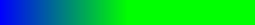| | `[0, 255]` |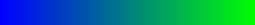| | `[127, 255]` |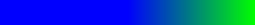| The values in the array must increase sequentially; for example, `[0, 63, 127, 190, 255]`. A transformation matrix as defined by the openfl.geom.Matrix class. The openfl.geom.Matrix class includes a `createGradientBox()` method, which lets you conveniently set up the matrix for use with the `beginGradientFill()` method. A value from the SpreadMethod class that specifies which spread method to use, either: `SpreadMethod.PAD`, `SpreadMethod.REFLECT`, or `SpreadMethod.REPEAT`. For example, consider a simple linear gradient between two colors: ``````import flash.geom.* import flash.display.* var fillType:String = GradientType.LINEAR; var colors:Array = [0xFF0000, 0x0000FF]; var alphas:Array = [1, 1]; var ratios:Array = [0x00, 0xFF]; var matr:Matrix = new Matrix(); matr.createGradientBox(20, 20, 0, 0, 0); var spreadMethod:String = SpreadMethod.PAD; this.graphics.beginGradientFill(fillType, colors, alphas, ratios, matr, spreadMethod); this.graphics.drawRect(0,0,100,100);`````` This example uses `SpreadMethod.PAD` for the spread method, and the gradient fill looks like the following: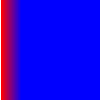If you use SpreadMethod.REFLECT for the spread method, the gradient fill looks like the following: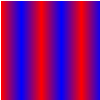If you use SpreadMethod.REPEAT for the spread method, the gradient fill looks like the following: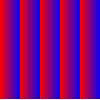A value from the InterpolationMethod class that specifies which value to use: `InterpolationMethod.LINEAR_RGB` or `InterpolationMethod.RGB` For example, consider a simple linear gradient between two colors (with the `spreadMethod` parameter set to `SpreadMethod.REFLECT`). The different interpolation methods affect the appearance as follows: | | | | --- | --- | |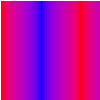`InterpolationMethod.LINEAR_RGB` |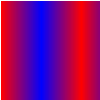`InterpolationMethod.RGB` | A number that controls the location of the focal point of the gradient. 0 means that the focal point is in the center. 1 means that the focal point is at one border of the gradient circle. -1 means that the focal point is at the other border of the gradient circle. A value less than -1 or greater than 1 is rounded to -1 or 1. For example, the following example shows a `focalPointRatio` set to 0.75: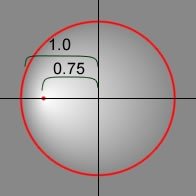Throws:

`ArgumentError` If the `type` parameter is not valid.

### `@:value({ matrix : null })beginShaderFill(shader:Shader, ?matrix:Matrix):Void`

Specifies a shader fill used by subsequent calls to other Graphics methods (such as `lineTo()` or `drawCircle()`) for the object. The fill remains in effect until you call the `beginFill()`, `beginBitmapFill()`, `beginGradientFill()`, or `beginShaderFill()` method. Calling the `clear()` method clears the fill. The application renders the fill whenever three or more points are drawn, or when the `endFill()` method is called.

Shader fills are not supported under GPU rendering; filled areas will be colored cyan.

Parameters:

`shader` The shader to use for the fill. This Shader instance is not required to specify an image input. However, if an image input is specified in the shader, the input must be provided manually. To specify the input, set the `input` property of the corresponding ShaderInput property of the `Shader.data` property. When you pass a Shader instance as an argument the shader is copied internally. The drawing fill operation uses that internal copy, not a reference to the original shader. Any changes made to the shader, such as changing a parameter value, input, or bytecode, are not applied to the copied shader that's used for the fill. A matrix object (of the openfl.geom.Matrix class), which you can use to define transformations on the shader. For example, you can use the following matrix to rotate a shader by 45 degrees (pi/4 radians): ``````matrix = new openfl.geom.Matrix(); matrix.rotate(Math.PI / 4);`````` The coordinates received in the shader are based on the matrix that is specified for the `matrix` parameter. For a default (`null`) matrix, the coordinates in the shader are local pixel coordinates which can be used to sample an input.

Throws:

`ArgumentError` When the shader output type is not compatible with this operation (the shader must specify a `pixel3` or `pixel4` output). When the shader specifies an image input that isn't provided. When a ByteArray or Vector. instance is used as an input and the `width` and `height` properties aren't specified for the ShaderInput, or the specified values don't match the amount of data in the input object. See the `ShaderInput.input` property for more information.

### `clear():Void`

Clears the graphics that were drawn to this Graphics object, and resets fill and line style settings.

### `copyFrom(sourceGraphics:Graphics):Void`

Copies all of drawing commands from the source Graphics object into the calling Graphics object.

Parameters:

`sourceGraphics` The Graphics object from which to copy the drawing commands.

### `cubicCurveTo(controlX1:Float, controlY1:Float, controlX2:Float, controlY2:Float, anchorX:Float, anchorY:Float):Void`

Draws a cubic Bezier curve from the current drawing position to the specified anchor point. Cubic Bezier curves consist of two anchor points and two control points. The curve interpolates the two anchor points and curves toward the two control points.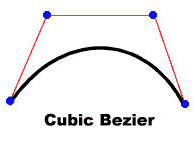The four points you use to draw a cubic Bezier curve with the `cubicCurveTo()` method are as follows:

• The current drawing position is the first anchor point.
• The `anchorX` and `anchorY` parameters specify the second anchor point.
• The `controlX1` and `controlY1` parameters specify the first control point.
• The `controlX2` and `controlY2` parameters specify the second control point.

If you call the `cubicCurveTo()` method before calling the `moveTo()` method, your curve starts at position (0, 0).

If the `cubicCurveTo()` method succeeds, the OpenFL runtime sets the current drawing position to (`anchorX`, `anchorY`). If the `cubicCurveTo()` method fails, the current drawing position remains unchanged.

If your movie clip contains content created with the Flash drawing tools, the results of calls to the `cubicCurveTo()` method are drawn underneath that content.

Parameters:

`controlX1` Specifies the horizontal position of the first control point relative to the registration point of the parent display object. Specifies the vertical position of the first control point relative to the registration point of the parent display object. Specifies the horizontal position of the second control point relative to the registration point of the parent display object. Specifies the vertical position of the second control point relative to the registration point of the parent display object. Specifies the horizontal position of the anchor point relative to the registration point of the parent display object. Specifies the vertical position of the anchor point relative to the registration point of the parent display object.

### `curveTo(controlX:Float, controlY:Float, anchorX:Float, anchorY:Float):Void`

Draws a quadratic curve using the current line style from the current drawing position to(anchorX, anchorY) and using the control point that (`controlX`, `controlY`) specifies. The current drawing position is then set to(`anchorX`, `anchorY`). If the movie clip in which you are drawing contains content created with the Flash drawing tools, calls to the `curveTo()` method are drawn underneath this content. If you call the `curveTo()` method before any calls to the `moveTo()` method, the default of the current drawing position is(0, 0). If any of the parameters are missing, this method fails and the current drawing position is not changed.

The curve drawn is a quadratic Bezier curve. Quadratic Bezier curves consist of two anchor points and one control point. The curve interpolates the two anchor points and curves toward the control point.Parameters:

`controlX` A number that specifies the horizontal position of the control point relative to the registration point of the parent display object. A number that specifies the vertical position of the control point relative to the registration point of the parent display object. A number that specifies the horizontal position of the next anchor point relative to the registration point of the parent display object. A number that specifies the vertical position of the next anchor point relative to the registration point of the parent display object.

### `drawEllipse(x:Float, y:Float, width:Float, height:Float):Void`

Draws an ellipse. Set the line style, fill, or both before you call the `drawEllipse()` method, by calling the `linestyle()`, `lineGradientStyle()`, `beginFill()`, `beginGradientFill()`, or `beginBitmapFill()` method.

Parameters:

`x` The x location of the top-left of the bounding-box of the ellipse relative to the registration point of the parent display object(in pixels). The y location of the top left of the bounding-box of the ellipse relative to the registration point of the parent display object(in pixels). The width of the ellipse(in pixels). The height of the ellipse(in pixels).

### `drawGraphicsData(graphicsData:Vector<IGraphicsData>):Void`

Submits a series of IGraphicsData instances for drawing. This method accepts a Vector containing objects including paths, fills, and strokes that implement the IGraphicsData interface. A Vector of IGraphicsData instances can refer to a part of a shape, or a complex fully defined set of data for rendering a complete shape.

Graphics paths can contain other graphics paths. If the `graphicsData` Vector includes a path, that path and all its sub-paths are rendered during this operation.

### `@:value({ winding : GraphicsPathWinding.EVEN_ODD })drawPath(commands:Vector<Int>, data:Vector<Float>, winding:GraphicsPathWinding = GraphicsPathWinding.EVEN_ODD):Void`

Submits a series of commands for drawing. The `drawPath()` method uses vector arrays to consolidate individual `moveTo()`, `lineTo()`, and `curveTo()` drawing commands into a single call. The `drawPath()` method parameters combine drawing commands with x- and y-coordinate value pairs and a drawing direction. The drawing commands are values from the GraphicsPathCommand class. The x- and y-coordinate value pairs are Numbers in an array where each pair defines a coordinate location. The drawing direction is a value from the GraphicsPathWinding class.

Generally, drawings render faster with `drawPath()` than with a series of individual `lineTo()` and `curveTo()` methods.

The `drawPath()` method uses a uses a floating computation so rotation and scaling of shapes is more accurate and gives better results. However, curves submitted using the `drawPath()` method can have small sub-pixel alignment errors when used in conjunction with the `lineTo()` and `curveTo()` methods.

The `drawPath()` method also uses slightly different rules for filling and drawing lines. They are:

• When a fill is applied to rendering a path: A sub-path of less than 3 points is not rendered.(But note that the stroke rendering will still occur, consistent with the rules for strokes below.) A sub-path that isn't closed(the end point is not equal to the begin point) is implicitly closed.
• When a stroke is applied to rendering a path: The sub-paths can be composed of any number of points. The sub-path is never implicitly closed.

Parameters:

`commands` A Vector of integers representing drawing commands. The set of accepted values is defined by the constants in the GraphicsPathCommand class. A Vector of Number instances where each pair of numbers is treated as a coordinate location (an x, y pair). The x- and y-coordinate value pairs are not Point objects; the data vector is a series of numbers where each group of two numbers represents a coordinate location. Specifies the winding rule using a value defined in the GraphicsPathWinding class.

### `@:value({ transforms : null, indices : null })drawQuads(rects:Vector<Float>, ?indices:Vector<Int>, ?transforms:Vector<Float>):Void`

Renders a set of quadrilaterals. This is similar to calling `drawRect` repeatedly, but each rectangle can use a transform value to rotate, scale or skew the result.

Any type of fill can be used, but if the fill has a transform matrix that transform matrix is ignored.

The optional `indices` parameter allows the use of either repeated rectangle geometry, or allows the use of a subset of a broader rectangle data Vector, such as Tileset `rectData`.

Parameters:

`rects` A Vector containing rectangle coordinates in [ x0, y0, width0, height0, x1, y1 ... ] format. A Vector containing optional index values to reference the data contained in `rects`. Each index is a rectangle index in the Vector, not an array index. If this parameter is ommitted, each index from `rects` will be used in order. A Vector containing optional transform data to adjust x, y, a, b, c or d value for the resulting quadrilateral. A `transforms` Vector that is double the size of the draw count (the length of `indices`, or if omitted, the rectangle count in `rects`) will be treated as [ x, y, ... ] pairs. A `transforms` Vector that is four times the size of the draw count will be used as matrix [ a, b, c, d, ... ] values. A `transforms` object which is six times the draw count in size will use full matrix [ a, b, c, d, tx, ty, ... ] values per draw.

### `drawRect(x:Float, y:Float, width:Float, height:Float):Void`

Draws a rectangle. Set the line style, fill, or both before you call the `drawRect()` method, by calling the `linestyle()`, `lineGradientStyle()`, `beginFill()`, `beginGradientFill()`, or `beginBitmapFill()` method.

Parameters:

`x` A number indicating the horizontal position relative to the registration point of the parent display object(in pixels). A number indicating the vertical position relative to the registration point of the parent display object(in pixels). The width of the rectangle(in pixels). The height of the rectangle(in pixels).

Throws:

`ArgumentError` If the `width` or `height` parameters are not a number `````` (`Number.NaN`). ``````

### `@:value({ ellipseHeight : null })drawRoundRect(x:Float, y:Float, width:Float, height:Float, ellipseWidth:Float, ?ellipseHeight:Float):Void`

Draws a rounded rectangle. Set the line style, fill, or both before you call the `drawRoundRect()` method, by calling the `linestyle()`, `lineGradientStyle()`, `beginFill()`, `beginGradientFill()`, or `beginBitmapFill()` method.

Parameters:

`x` A number indicating the horizontal position relative to the registration point of the parent display object(in pixels). A number indicating the vertical position relative to the registration point of the parent display object `````` (in pixels). `````` The width of the round rectangle(in pixels). The height of the round rectangle(in pixels). The width of the ellipse used to draw the rounded corners(in pixels). The height of the ellipse used to draw the rounded corners(in pixels). Optional; if no value is specified, the default value matches that provided for the `ellipseWidth` parameter.

Throws:

`ArgumentError` If the `width`, `height`, `ellipseWidth` or `ellipseHeight` parameters are not a number(`Number.NaN`).

### `drawRoundRectComplex(x:Float, y:Float, width:Float, height:Float, topLeftRadius:Float, topRightRadius:Float, bottomLeftRadius:Float, bottomRightRadius:Float):Void`

Undocumented method

### `@:value({ culling : TriangleCulling.NONE, uvtData : null, indices : null })drawTriangles(vertices:Vector<Float>, ?indices:Vector<Int>, ?uvtData:Vector<Float>, culling:TriangleCulling = TriangleCulling.NONE):Void`

Renders a set of triangles, typically to distort bitmaps and give them a three-dimensional appearance. The `drawTriangles()` method maps either the current fill, or a bitmap fill, to the triangle faces using a set of(u,v) coordinates.

Any type of fill can be used, but if the fill has a transform matrix that transform matrix is ignored.

A `uvtData` parameter improves texture mapping when a bitmap fill is used.

Parameters:

`culling` Specifies whether to render triangles that face in a specified direction. This parameter prevents the rendering of triangles that cannot be seen in the current view. This parameter can be set to any value defined by the TriangleCulling class.

### `endFill():Void`

Applies a fill to the lines and curves that were added since the last call to the `beginFill()`, `beginGradientFill()`, or `beginBitmapFill()` method. Flash uses the fill that was specified in the previous call to the `beginFill()`, `beginGradientFill()`, or `beginBitmapFill()` method. If the current drawing position does not equal the previous position specified in a `moveTo()` method and a fill is defined, the path is closed with a line and then filled.

### `@:value({ smooth : false, repeat : true, matrix : null })lineBitmapStyle(bitmap:BitmapData, ?matrix:Matrix, repeat:Bool = true, smooth:Bool = false):Void`

Specifies a bitmap to use for the line stroke when drawing lines.

The bitmap line style is used for subsequent calls to Graphics methods such as the `lineTo()` method or the `drawCircle()` method. The line style remains in effect until you call the `lineStyle()` or `lineGradientStyle()` methods, or the `lineBitmapStyle()` method again with different parameters.

You can call the `lineBitmapStyle()` method in the middle of drawing a path to specify different styles for different line segments within a path.

Call the `lineStyle()` method before you call the `lineBitmapStyle()` method to enable a stroke, or else the value of the line style is `undefined`.

Calls to the `clear()` method set the line style back to `undefined`.

Parameters:

`bitmap` The bitmap to use for the line stroke. An optional transformation matrix as defined by the openfl.geom.Matrix class. The matrix can be used to scale or otherwise manipulate the bitmap before applying it to the line style. Whether to repeat the bitmap in a tiled fashion. Whether smoothing should be applied to the bitmap.

### `@:value({ focalPointRatio : 0, interpolationMethod : InterpolationMethod.RGB, spreadMethod : SpreadMethod.PAD, matrix : null })lineGradientStyle(type:GradientType, colors:Array<Int>, alphas:Array<Float>, ratios:Array<Int>, ?matrix:Matrix, spreadMethod:SpreadMethod = SpreadMethod.PAD, interpolationMethod:InterpolationMethod = InterpolationMethod.RGB, focalPointRatio:Float = 0):Void`

Specifies a gradient to use for the stroke when drawing lines.

The gradient line style is used for subsequent calls to Graphics methods such as the `lineTo()` methods or the `drawCircle()` method. The line style remains in effect until you call the `lineStyle()` or `lineBitmapStyle()` methods, or the `lineGradientStyle()` method again with different parameters.

You can call the `lineGradientStyle()` method in the middle of drawing a path to specify different styles for different line segments within a path.

Call the `lineStyle()` method before you call the `lineGradientStyle()` method to enable a stroke, or else the value of the line style is `undefined`.

Calls to the `clear()` method set the line style back to `undefined`.

Parameters:

`type` A value from the GradientType class that specifies which gradient type to use: `GradientType.LINEAR` or `GradientType.RADIAL`. An array of RGB hex color values to be used in the gradient (for example, red is 0xFF0000, blue is 0x0000FF, and so on). An array of alpha values for the corresponding colors in the `colors` array; valid values are 0 to 1. If the value is less than 0, the default is 0. If the value is greater than 1, the default is 1. An array of color distribution ratios; valid values are from 0 to 255. This value defines the percentage of the width where the color is sampled at 100%. The value 0 represents the left position in the gradient box, and 255 represents the right position in the gradient box. This value represents positions in the gradient box, not the coordinate space of the final gradient, which can be wider or thinner than the gradient box. Specify a value for each value in the `colors` parameter. For example, for a linear gradient that includes two colors, blue and green, the following figure illustrates the placement of the colors in the gradient based on different values in the `ratios` array: | ratios | Gradient | | --- | --- | | `[0, 127]` || | `[0, 255]` || | `[127, 255]` || The values in the array must increase sequentially; for example, `[0, 63, 127, 190, 255]`. A transformation matrix as defined by the openfl.geom.Matrix class. The openfl.geom.Matrix class includes a `createGradientBox()` method, which lets you conveniently set up the matrix for use with the `lineGradientStyle()` method. A value from the SpreadMethod class that specifies which spread method to use: | | | | | --- | --- | --- | |`SpreadMethod.PAD` |`SpreadMethod.REFLECT` |`SpreadMethod.REPEAT` | A value from the InterpolationMethod class that specifies which value to use. For example, consider a simple linear gradient between two colors (with the `spreadMethod` parameter set to `SpreadMethod.REFLECT`). The different interpolation methods affect the appearance as follows: | | | | --- | --- | |`InterpolationMethod.LINEAR_RGB` |`InterpolationMethod.RGB` | A number that controls the location of the focal point of the gradient. The value 0 means the focal point is in the center. The value 1 means the focal point is at one border of the gradient circle. The value -1 means that the focal point is at the other border of the gradient circle. Values less than -1 or greater than 1 are rounded to -1 or 1. The following image shows a gradient with a `focalPointRatio` of -0.75:### `@:value({ miterLimit : 3, joints : null, caps : null, scaleMode : LineScaleMode.NORMAL, pixelHinting : false, alpha : 1, color : 0, thickness : null })lineStyle(?thickness:Float, color:Int = 0, alpha:Float = 1, pixelHinting:Bool = false, scaleMode:LineScaleMode = LineScaleMode.NORMAL, ?caps:CapsStyle, ?joints:JointStyle, miterLimit:Float = 3):Void`

Specifies a line style used for subsequent calls to Graphics methods such as the `lineTo()` method or the `drawCircle()` method. The line style remains in effect until you call the `lineGradientStyle()` method, the `lineBitmapStyle()` method, or the `lineStyle()` method with different parameters.

You can call the `lineStyle()` method in the middle of drawing a path to specify different styles for different line segments within the path.

Note: Calls to the `clear()` method set the line style back to `undefined`.

Note: Flash Lite 4 supports only the first three parameters (`thickness`, `color`, and `alpha`).

Parameters:

`thickness`

An integer that indicates the thickness of the line in points; valid values are 0-255. If a number is not specified, or if the parameter is undefined, a line is not drawn. If a value of less than 0 is passed, the default is 0. The value 0 indicates hairline thickness; the maximum thickness is 255. If a value greater than 255 is passed, the default is 255.

`color`

A hexadecimal color value of the line; for example, red is 0xFF0000, blue is 0x0000FF, and so on. If a value is not indicated, the default is 0x000000

``````			   (black). Optional.
``````
`alpha`

A number that indicates the alpha value of the color of the line; valid values are 0 to 1. If a value is not indicated, the default is 1(solid). If the value is less than 0, the default is 0. If the value is greater than 1, the default is 1.

`pixelHinting`

(Not supported in Flash Lite 4) A Boolean value that specifies whether to hint strokes to full pixels. This affects both the position of anchors of a curve and the line stroke size itself. With `pixelHinting` set to `true`, line widths are adjusted to full pixel widths. With `pixelHinting` set to `false`, disjoints can appear for curves and straight lines. For example, the following illustrations show how Flash Player or Adobe AIR renders two rounded rectangles that are identical, except that the `pixelHinting` parameter used in the `lineStyle()` method is set differently

``````			   (the images are scaled by 200%, to emphasize the
``````

difference):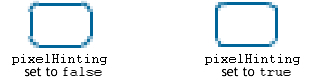If a value is not supplied, the line does not use pixel hinting.

`scaleMode`

(Not supported in Flash Lite 4) A value from the LineScaleMode class that specifies which scale mode to use:

• `LineScaleMode.NORMAL` - Always scale the line thickness when the object is scaled

``````		   (the default).
``````
• `LineScaleMode.NONE` - Never scale the line thickness.
• `LineScaleMode.VERTICAL` - Do not scale the line thickness if the object is scaled vertically only. For example, consider the following circles, drawn with a one-pixel line, and each with the `scaleMode` parameter set to `LineScaleMode.VERTICAL`. The circle on the left is scaled vertically only, and the circle on the right is scaled both vertically and horizontally: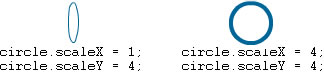• `LineScaleMode.HORIZONTAL` - Do not scale the line thickness if the object is scaled horizontally only. For example, consider the following circles, drawn with a one-pixel line, and each with the `scaleMode` parameter set to `LineScaleMode.HORIZONTAL`. The circle on the left is scaled horizontally only, and the circle on the right is scaled both vertically and horizontally: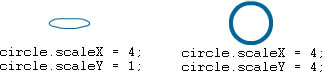`caps`

(Not supported in Flash Lite 4) A value from the CapsStyle class that specifies the type of caps at the end of lines. Valid values are: `CapsStyle.NONE`, `CapsStyle.ROUND`, and `CapsStyle.SQUARE`. If a value is not indicated, Flash uses round caps.

For example, the following illustrations show the different `capsStyle` settings. For each setting, the illustration shows a blue line with a thickness of 30(for which the `capsStyle` applies), and a superimposed black line with a thickness of 1(for which no `capsStyle` applies):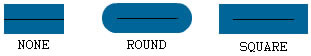`joints`

(Not supported in Flash Lite 4) A value from the JointStyle class that specifies the type of joint appearance used at angles. Valid values are: `JointStyle.BEVEL`, `JointStyle.MITER`, and `JointStyle.ROUND`. If a value is not indicated, Flash uses round joints.

For example, the following illustrations show the different `joints` settings. For each setting, the illustration shows an angled blue line with a thickness of 30(for which the `jointStyle` applies), and a superimposed angled black line with a thickness of 1(for which no `jointStyle` applies):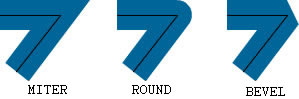Note: For `joints` set to `JointStyle.MITER`, you can use the `miterLimit` parameter to limit the length of the miter.

`miterLimit`

(Not supported in Flash Lite 4) A number that indicates the limit at which a miter is cut off. Valid values range from 1 to 255(and values outside that range are rounded to 1 or 255). This value is only used if the `jointStyle` is set to `"miter"`. The `miterLimit` value represents the length that a miter can extend beyond the point at which the lines meet to form a joint. The value expresses a factor of the line `thickness`. For example, with a `miterLimit` factor of 2.5 and a `thickness` of 10 pixels, the miter is cut off at 25 pixels.

For example, consider the following angled lines, each drawn with a `thickness` of 20, but with `miterLimit` set to 1, 2, and 4. Superimposed are black reference lines showing the meeting points of the joints: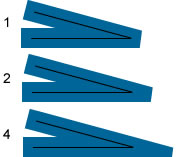Notice that a given `miterLimit` value has a specific maximum angle for which the miter is cut off. The following table lists some examples:

miterLimit value:Angles smaller than this are cut off:
1.41490 degrees
260 degrees
430 degrees
815 degrees

### `lineTo(x:Float, y:Float):Void`

Draws a line using the current line style from the current drawing position to(`x`, `y`); the current drawing position is then set to(`x`, `y`). If the display object in which you are drawing contains content that was created with the Flash drawing tools, calls to the `lineTo()` method are drawn underneath the content. If you call `lineTo()` before any calls to the `moveTo()` method, the default position for the current drawing is(0, 0). If any of the parameters are missing, this method fails and the current drawing position is not changed.

Parameters:

`x` A number that indicates the horizontal position relative to the registration point of the parent display object(in pixels). A number that indicates the vertical position relative to the registration point of the parent display object(in pixels).

### `moveTo(x:Float, y:Float):Void`

Moves the current drawing position to(`x`, `y`). If any of the parameters are missing, this method fails and the current drawing position is not changed.

Parameters:

`x` A number that indicates the horizontal position relative to the registration point of the parent display object(in pixels). A number that indicates the vertical position relative to the registration point of the parent display object(in pixels).

### `@:value({ recurse : true })readGraphicsData(recurse:Bool = true):Vector<IGraphicsData>`

Queries a Sprite or Shape object (and optionally, its children) for its vector graphics content. The result is a Vector of IGraphicsData objects. Transformations are applied to the display object before the query, so the returned paths are all in the same coordinate space. Coordinates in the result data set are relative to the stage, not the display object being sampled.

The result includes the following types of objects, with the specified limitations:

• GraphicsSolidFill
• GraphicsGradientFill All properties of the gradient fill are returned by `readGraphicsData()`. The matrix returned is close to, but not exactly the same as, the input matrix.
• GraphicsEndFill
• GraphicsBitmapFill The matrix returned is close to, but not exactly the same as, the input matrix. `repeat` is always `true`. * `smooth` is always `false`.
• GraphicsStroke `thickness` is supported. `fill` supports GraphicsSolidFill, GraphicsGradientFill, and GraphicsBitmapFill as described previously * All other properties have default values.
• GraphicsPath * The only supported commands are `MOVE_TO`, `CURVE_TO`, and `LINE_TO`.

The following visual elements and transformations can't be represented and are not included in the result:

• Text, with one exception: Static text that is defined with anti-alias type "anti-alias for animation" is rendered as vector shapes so it is included in the result.
• Blend modes
• 9-slice scaling
• Triangles (created with the `drawTriangles()` method)
• Opaque background
• `scrollRect` settings
• 2.5D transformations
• Non-visible objects (objects whose `visible` property is `false`)

Parameters:

`recurse` whether the runtime should also query display object children of the current display object. A recursive query can take more time and memory to execute. The results are returned in a single flattened result set, not separated by display object.

Returns:

A Vector of IGraphicsData objects representing the vector graphics content of the related display object